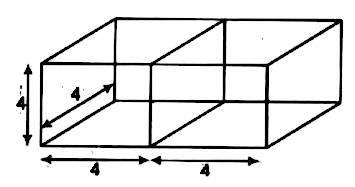Home/Class 10 Math Chapter List/15. Surface Areas and Volumes/

# 2 cubes each of volume (64;cm^{3}) are joined end to end. Find the surface area of the resulting cuboid.

## QuestionMathClass 10

2 cubes each of volume $$64\;cm^{3}$$ are joined end to end. Find the surface area of the resulting cuboid.

$$160\ cm^2$$
4.64.6## Solution

Given, the volume of a cube is $$64\;cm^{3}$$.Let side of the cube is $$a\;cm$$,then$$a^{3}=64=4^{3}$$
$$\Rightarrow$$$$a=4\;cm$$
When we join each cube end to end, then, length of the new cuboid become
$$(4+4)=8\;cm$$.
Width and Height of the cuboid is 4cm each.
$$\therefore$$The surface area of the resulting cuboid $$=2(lb+bh+hl)$$
$$=2(8\times 4+4\times 4+4\times 8)$$
$$=2(32+16+32)$$
$$=2(80)$$
$$=160\;cm^{2}$$
Hence, the total surface area of the cuboid is $$160cm^2$$.